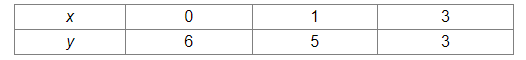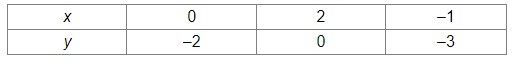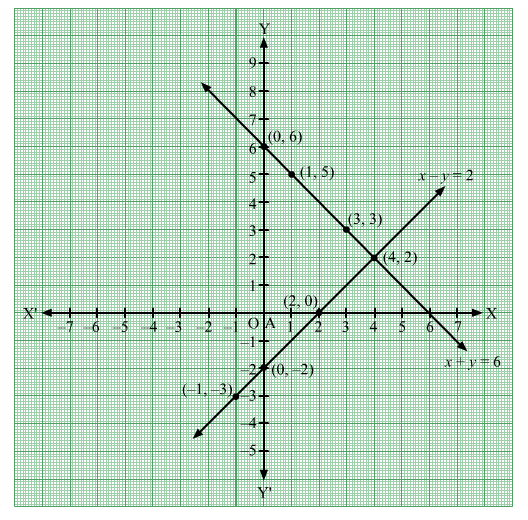# Draw the graph for each of the equation x + y = 6 and x – y = 2

Question:

Draw the graph for each of the equation x + y = 6 and x – y = 2 on the same graph paper and find the coordinates of the point where the two straight lines intersect.

Solution:

$x+y=6$

$\Rightarrow y=-x+6$

When $x=0, y=-0+6=6$

When $x=1, y=-1+6=5$

When $x=3, y=-3+6=3$

Thus, the points on the line x + y = 6 are as given in the following table:Plotting the points (0, 6), (1, 5) and (33) and drawing a line passing through these points, we obtain the graph of of the line x + y = 6.

$x-y=2$

$\Rightarrow y=x-2$

When $x=0, y=0-2=-2$

When $x=2, y=2-2=0$

When $x=-1, y=-1-2=-3$

Thus, the points on the line x – y = 2 are as given in the following table:Plotting the points (0, –2), (2, 0) and (–1–3) and drawing a line passing through these points, we obtain the graph of of the line x – y = 2.It can be seen that the lines x + y = 6 and x – y = 2 intersect at the point (4, 2).